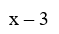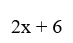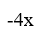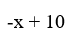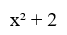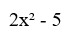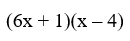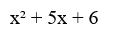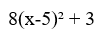# Linear vs Non-Linear Relations

## Differences between Linear and Quadratic Equations

• Qudratic functions are expressed as $$y = x^2$$ while linear expressions are expressed as $$y = x$$
• Quadratic functions have $$2$$ as the degree of their highest term whereas linear functions have $$1$$ as their highest degree
• All quadratic functions increase and decrease regradless of the slope whereas linear equations either always increase (if the slope is positive) or decrease (if the slope is negative)
• The slope of a quadratic function is constantly changing. The slope of a linear function is always constant
• Linear functions have each input produce a unique output. Conversely, quadratic functions (with the exception of the vertex) have pairs of unique independent variables produce the same output (ie $$x$$ values of $$2$$ and $$-2$$ would produce the same result)

Scatter plots can also be used to identify the different relations between $$x$$ and $$y$$:

• The relationship is considered linear when the points on a scatter plot follow a somewhat straight line pattern
• The relationship is considered non-linear if the points on a scatter plot follow a pattern but not a straight lineLinear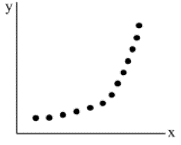Non-Linear

## Identifying Linear and Non-Linear Equations

As stated above, a function can be determined by the degree of their highest term - also known as the Leading Term (ie a Linear Function's highest term is $$1$$ while a Quadratic Function's highest term is $$2$$). You will learn about other non-linear relations if you continue studying math!

Try and fit the following functions into their correct description: